The goal of this blog post is to arrange a irregularly (with varying time intervals) spaced raster stack from Landsat into a regular time series to be used in the Breaks For Additive Season and Trend (`bfast`) package and function.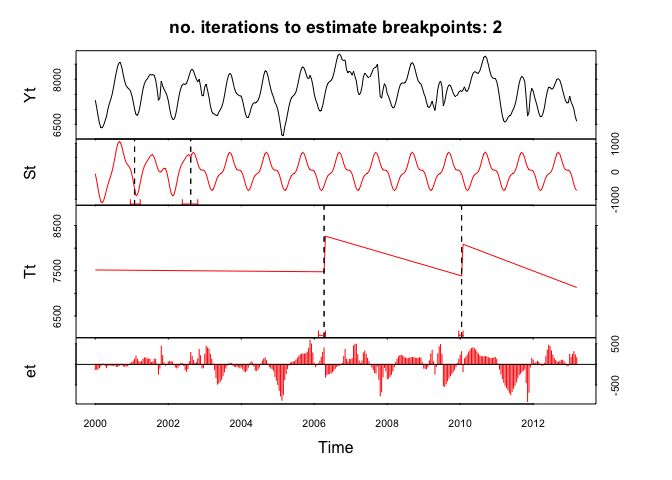BFAST plot generated with a time series of aggregated bi-weekly NDVI values.

# Objective

During the process we impute missing data (NA’s) either:

• linearly with `na.approx` from the `zoo` package, and/or
• periodic decomposition with `na.interp` from the `forecast` package.

We first create a regular daily time series and from that we aggregate the time series with the following frequencies:

• daily (frequency 365) - the base
• weekly (52)
• fortnightly (26)
• monthly (12)
• quarterly (4)
• yearly (1)

# Workflow

``````### R

library(zoo)
library(xts)
library(forecast)
library(seas)
library(bfast)
library(devtools)
install_github('loicdtx/bfastSpatial')
library(lubridate)
library(ggplot2)``````

## Load the data and select one example pixel

``````### R

data(tura)

# get the image dates
dates <- getZ(tura)

selected_pixel <- 90

# number of valid values in TS
nbr.NA <- sum(!is.na( !as.vector(tura[selected_pixel])))

# create a regular time series object by combining data and date information
(s <- bfastts(as.vector(tura[selected_pixel]), dates, type = c("irregular")))

## Time Series:
## Start = c(1984, 157)
## End = c(2013, 101)
## Frequency = 365
##        NA   NA   NA   NA   NA   NA   NA   NA   NA   NA   NA   NA   NA
##       NA   NA   NA   NA   NA   NA   NA   NA   NA   NA   NA   NA   NA
##       NA   NA   NA   NA   NA   NA   NA   NA   NA   NA   NA   NA   NA
##       NA   NA   NA   NA   NA   NA   NA   NA   NA   NA   NA   NA   NA
##       NA   NA   NA   NA   NA   NA   NA   NA   NA   NA   NA   NA   NA
##       NA   NA   NA   NA   NA   NA   NA   NA   NA   NA   NA   NA   NA
##       NA   NA   NA   NA   NA   NA   NA   NA   NA   NA   NA   NA   NA
##       NA   NA   NA   NA   NA   NA   NA   NA   NA   NA   NA   NA   NA
##      NA   NA   NA   NA   NA   NA   NA   NA   NA   NA   NA   NA   NA
##      NA   NA   NA   NA   NA   NA   NA   NA   NA   NA   NA 7388   NA
##      NA   NA   NA   NA   NA   NA   NA   NA   NA   NA   NA   NA   NA
##      NA   NA   NA   NA   NA   NA   NA   NA   NA   NA   NA   NA   NA
##      NA   NA   NA   NA 5886   NA   NA   NA   NA   NA   NA   NA   NA
##      NA   NA   NA   NA   NA   NA   NA   NA   NA   NA   NA   NA   NA
##      NA   NA   NA   NA   NA   NA   NA   NA   NA   NA   NA   NA   NA
##      NA   NA   NA   NA   NA   NA   NA   NA   NA   NA   NA   NA   NA
##    6752   NA   NA   NA   NA   NA   NA   NA   NA   NA   NA   NA   NA
##
##   ....
##
##    NA   NA   NA   NA   NA   NA   NA   NA   NA   NA   NA   NA   NA
##    NA   NA   NA   NA   NA   NA   NA   NA   NA   NA   NA   NA   NA
##    NA 7176   NA   NA   NA   NA   NA   NA   NA   NA   NA   NA   NA
##    NA   NA   NA   NA 7526   NA   NA   NA   NA   NA   NA   NA   NA
##    NA   NA   NA   NA   NA   NA   NA 7112   NA   NA   NA   NA   NA
##    NA   NA   NA   NA   NA   NA   NA   NA   NA   NA 7154   NA   NA
##    NA   NA   NA   NA   NA   NA   NA   NA   NA   NA   NA   NA   NA
##    NA   NA   NA   NA   NA   NA   NA   NA   NA   NA   NA   NA   NA
##    NA   NA   NA   NA   NA   NA   NA   NA   NA   NA   NA   NA   NA
##    NA   NA   NA   NA   NA   NA 6576   NA   NA   NA   NA   NA   NA
##    NA   NA   NA   NA   NA   NA   NA   NA   NA   NA   NA   NA   NA
##    NA   NA   NA   NA   NA   NA   NA   NA   NA   NA   NA   NA 7444

# First day of the Time Series
##  "1984-06-05 10:15:27 UTC"

# Last day of the Time Series
(last.day <- tail(date_decimal(as.numeric(time(s))),1))
##  "2013-04-10 23:59:59 UTC"``````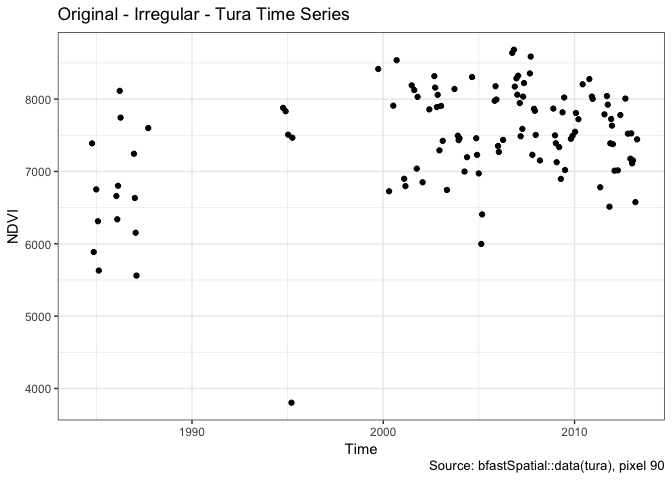## Create Daily Time Series (NA’s - linear, periodic)

Replace NA’s by Linear Interpolation with `na.approx` from the `zoo` package

``````### R

s.d.linear <- round(na.approx(s),0)

# convert the time series to a data.frame
time.series.to.dataframe <- function(time_series, source) {

s.df           <- data.frame(as.numeric(time_series))
colnames(s.df) <- "NDVI"
s.df\$Time      <- as.Date(date_decimal(as.numeric(time(time_series))))
s.df\$Type      <- source
return(s.df)

}

# create a data.frame
s.d.linear.df <- time.series.to.dataframe(s.d.linear, "Linear Interpolation")``````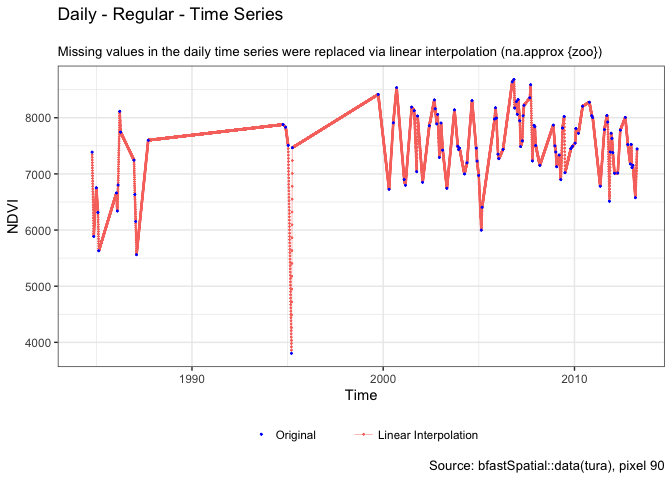Replace NA’s by periodic stl decomposition with `na.interp` from the `forecast` package

``````### R

s.d.periodic <- round(na.interp(s),0)``````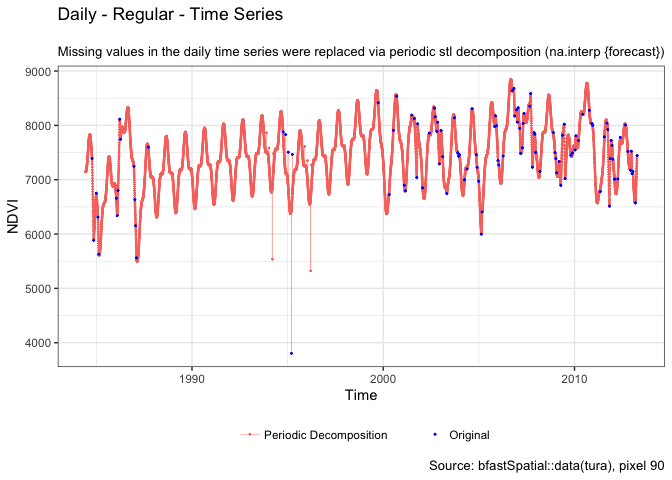### Aggregate Time Series from Daily to Weekly

``````### R

aggregate.daily.to.weekly <- function(daily.ts) {

dates      <- as.Date(date_decimal(as.numeric(time(daily.ts))))

xts.daily  <- xts(daily.ts, order.by = dates)

xts.weekly <- round(xts::apply.weekly(xts.daily, median),0)  # xts

start(xts.weekly)
ts.weekly <- ts(data = xts.weekly,
# define the start and end (Year, Week)
start = c(as.numeric(format(start(xts.weekly), "%Y")),
as.numeric(format(start(xts.weekly), "%W"))),
end   = c(as.numeric(format(end(xts.weekly), "%Y")),
as.numeric(format(end(xts.weekly), "%W"))),
frequency = 52)

return(ts.weekly)
}

s.w.linear   <- aggregate.daily.to.weekly(s.d.linear)

start(s.w.linear)
##  1984   41

end(s.w.linear)
##  2013   14

s.w.periodic   <- aggregate.daily.to.weekly(s.d.periodic)``````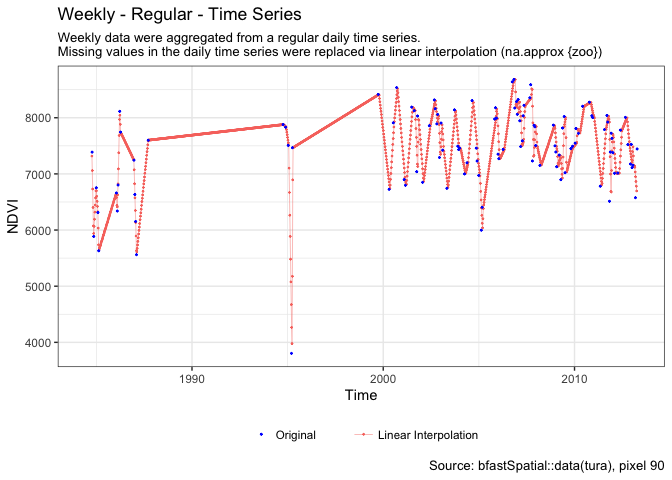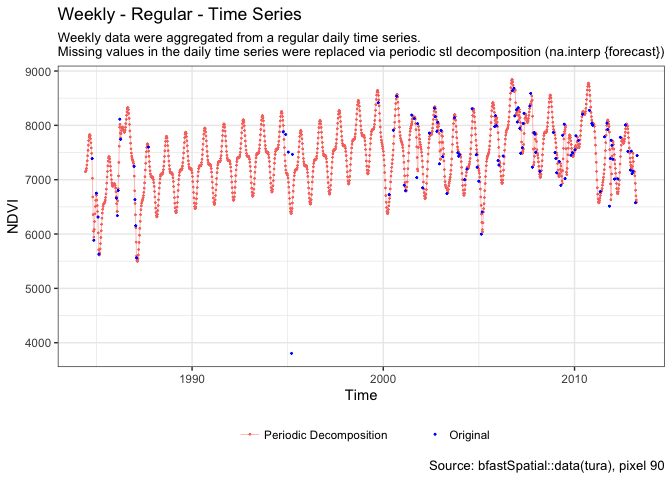### Aggregate Time Series from Daily to Fortnightly

``````### R

aggregate.daily.to.fortnight <- function(daily.ts) {

daily.ts.df           <- data.frame(as.numeric(daily.ts))
colnames(daily.ts.df) <- "NDVI"
daily.ts.df\$date      <- as.Date(date_decimal(as.numeric(time(daily.ts))))

library(openair)
## bi-weekly / fortnightly averages with function timeAverage {openair}
fortnight      <- timeAverage(daily.ts.df, avg.time = "2 week")
fortnight\$time <- as.Date(fortnight\$date)

fortnight.ts <- ts(fortnight\$NDVI,
# define the start and end (Year, Week)
start = c(as.numeric(format(min(fortnight\$date), "%Y")),
round(yday(min(fortnight\$date)) / 14,0)), # DOY / 14
end   = c(as.numeric(format(max(fortnight\$date), "%Y")),
round(yday(max(fortnight\$date)) / 14,0)), # DOY / 14
frequency = 26)

return(fortnight.ts)
}

s.f.linear   <- aggregate.daily.to.fortnight(s.d.linear)
## Warning: package 'openair' was built under R version 3.4.4

s.f.periodic <- aggregate.daily.to.fortnight(s.d.periodic)

start(s.f.linear)
##  1984   20

end(s.f.linear)
##  2013    6``````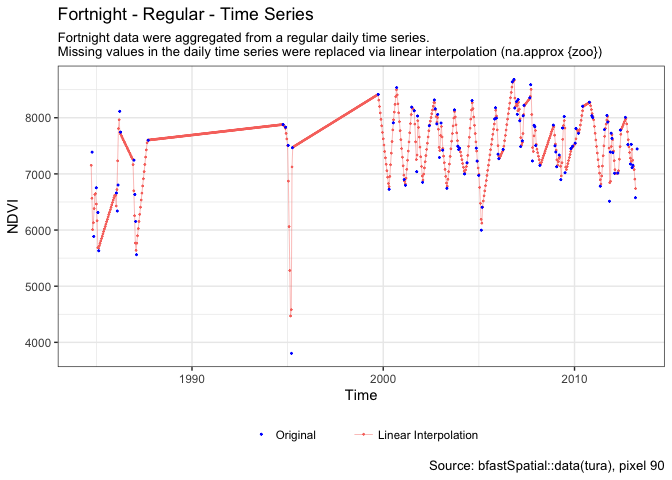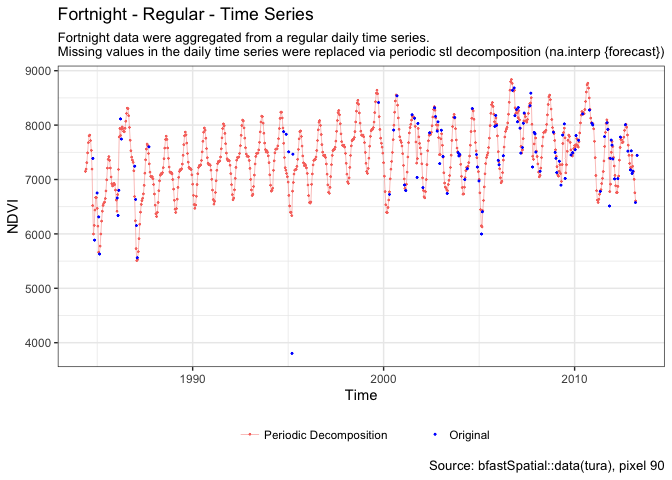### Aggregate Time Series from Daily to Monthly

``````### R

aggregate.daily.to.monthly <- function(daily.ts) {

s.month <- round(aggregate(as.zoo(daily.ts), as.yearmon, median), 0)   # zoo
s.month <- as.ts(s.month)

return(s.month)

}

s.m.linear   <- aggregate.daily.to.monthly(s.d.linear)
s.m.periodic <- aggregate.daily.to.monthly(s.d.periodic)

start(s.m.linear)
##  1984   10

end(s.m.linear)
##  2013    4``````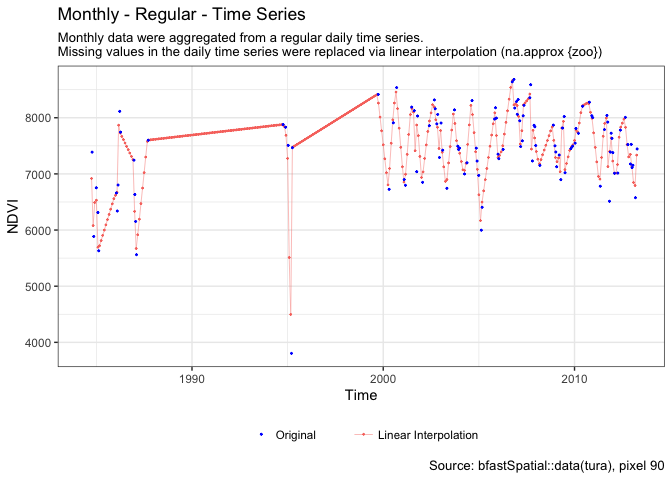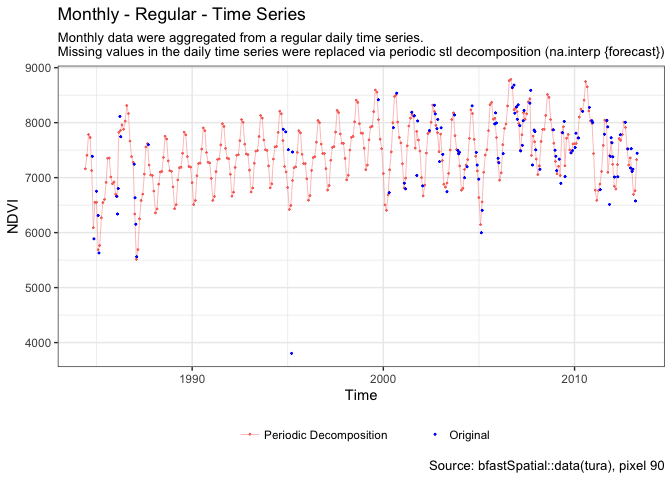### Aggregate Time Series from Daily to Quarterly

``````### R

aggregate.daily.to.quarterly <- function(daily.ts) {

s.qtr <- round(aggregate(as.zoo(daily.ts), as.yearqtr, median), 0)   # zoo
s.qtr <- as.ts(s.qtr)

return(s.qtr)

}

s.q.linear   <- aggregate.daily.to.quarterly(s.d.linear)
s.q.periodic <- aggregate.daily.to.quarterly(s.d.periodic)

start(s.q.linear)
##  1984    4

end(s.q.linear)
##  2013    2``````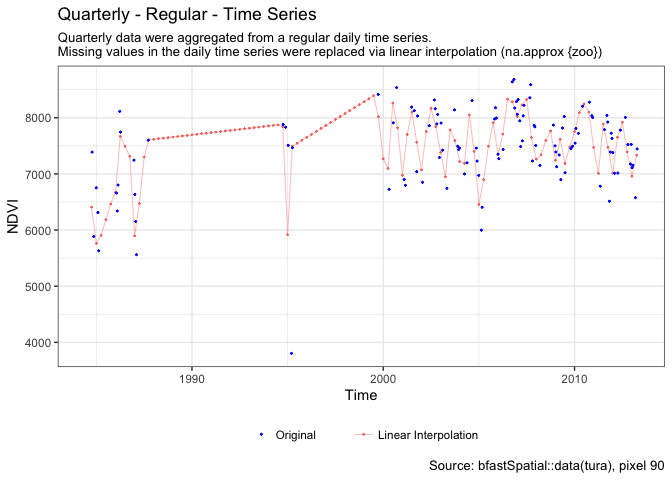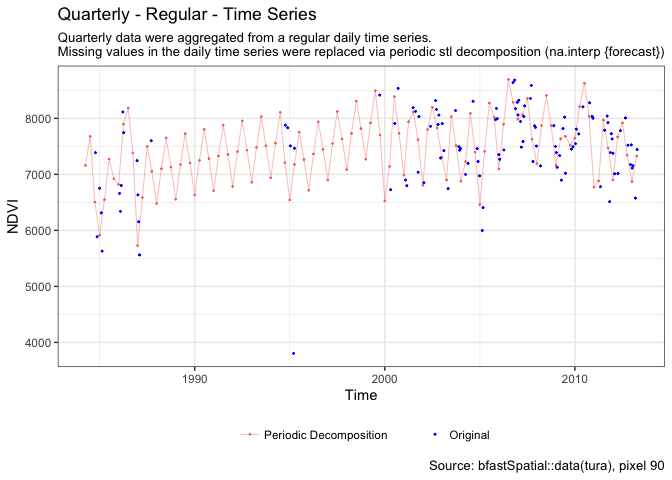### Aggregate Time Series from Daily to Yearly Season

The time of summer start and end depends on the location on the globe. The Tura dataset is from Ethopia and we consider June, July and August as their summer season, meaning we consider only these months for our yearly aggragation calculation.

``````### R

#https://stackoverflow.com/questions/18978256/what-is-the-most-elegant-way-to-calculate-seasonal-means-with-r

aggregate.daily.to.yearly <- function(daily.ts) {

# create data.frame
daily.df <- time.series.to.dataframe(daily.ts, "not needed here")

daily.df\$year <- format(daily.df\$Time, "%Y")

daily.df\$seas <- mkseas(x = daily.df, width = "DJF")

# calculate median per season within each year
year.df <- aggregate(NDVI ~ seas + year, data = daily.df, median)

# based on variable values
year.df.JJA <- year.df[ which(year.df\$seas == 'JJA'), ]

# create ts
year.ts.JJA <- ts(year.df.JJA\$NDVI,
start = c(as.numeric(min(year.df.JJA\$year)), 1), # freq 1
end   = c(as.numeric(max(year.df.JJA\$year)), 1), # freq 1
frequency = 1)

return(year.ts.JJA)
}

(s.y.linear   <- aggregate.daily.to.yearly(s.d.linear))
## Time Series:
## Start = 1985
## End = 2012
## Frequency = 1
##   6088.5 7552.0 7012.5 7633.0 7672.0 7712.0 7751.0 7791.0 7830.0 7870.0
##  7527.0 7739.5 7950.5 8162.0 8373.5 7963.0 8133.5 8080.5 7476.0 7872.0
##  7286.5 8116.5 8298.5 7509.5 7187.0 8227.5 7656.5 7904.0

(s.y.periodic <- aggregate.daily.to.yearly(s.d.periodic))
## Time Series:
## Start = 1984
## End = 2012
## Frequency = 1
##   7433.0 6914.5 8028.0 7063.5 7367.5 7443.5 7519.5 7595.5 7671.5 7747.5
##  7824.0 7460.0 7645.0 7829.0 8015.0 8200.0 7997.5 8142.5 8008.0 7501.5
##  7715.0 7853.5 8304.0 8161.0 8132.0 7629.5 8409.0 7585.5 7784.5``````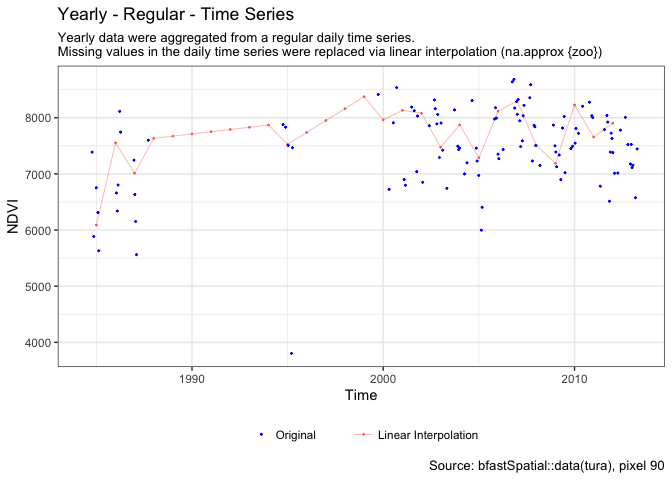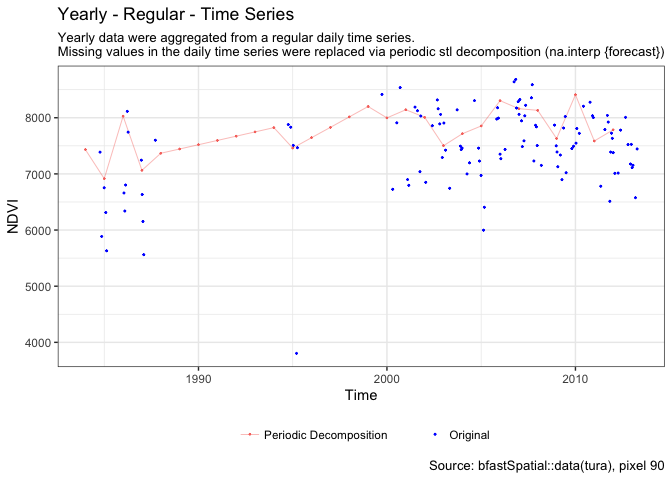## Focus on a Specific Period

Once you have your data prepared and you would like to work on a specific temporal window you can use the ‘window’ function to cookie cut the period of interest.

For example, use the Fortnight time series and focus on the data from year 2000, onwards.

``````### R

s.f.periodic.window <- window(x = s.f.periodic,
start = c(2000,1),
frequency = 26) # Fortnight has a frequency of 26

plot(s.f.periodic.window, ylab = "NDVI")``````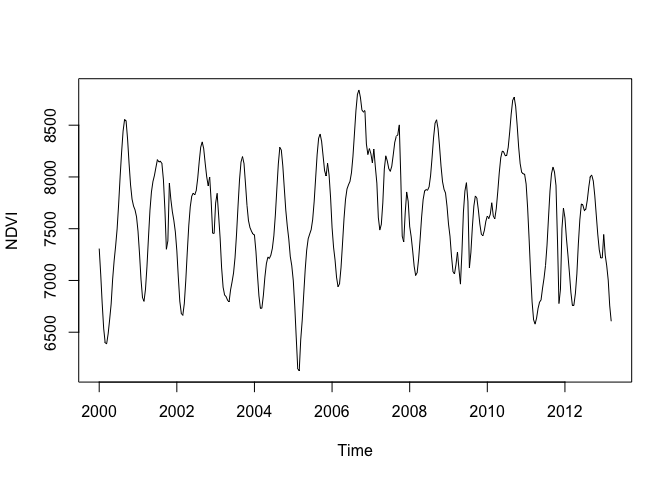### Testing the aggregated Time Series with BFAST

Here we do a simple test if `bfast` will accept the created fortnight time series. Lets see…

Yeap … it works beautifully!

``````### R

fit <- bfast(s.f.periodic.window, h = 10/length(s.f.periodic.window),
season = "harmonic", breaks = 2, max.iter = 2)

plot(fit)``````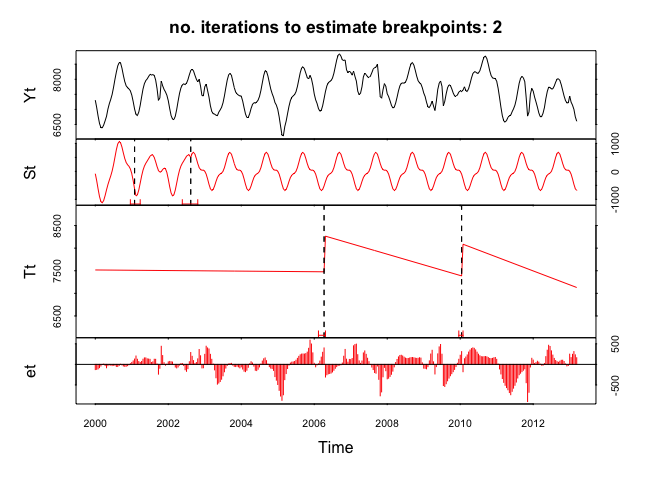# Bottom line

This blog post showed you how to arrange and aggragate a raster image stack in order to create a regular time series which can be used with the bfast function. If you have any questions, suggestions or spotted a mistake, please use the comment function at the bottom of this page.

You can cite this blogpost using: Gärtner, Philipp (2018/04/16) “How to arrange a raster image stack for the use with BFAST in R”, https://philippgaertner.github.io/2018/04/bfast-preparation/

Previous blog posts are available within the blog archive. Feel free to connect or follow me on Twitter - @Mixed_Pixels.

# Data Source

• Tura: The used Tura dataset is provided by the `bfastSpatial` package. It covers a area in Tura kebele, Kafa Zone, SW Ethiopia. The values are NDVI rescaled by a factor of 10000. Data originate from the Landsat 5 TM and Landsat 7 ETM+ sensors. Original Landsat scene names can be found by typing `names(tura)`. Dates are also contained in the z-dimension `getZ(tura)`.